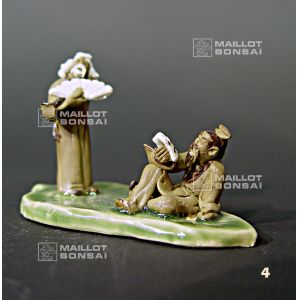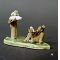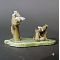##### The Japanese Bonsai specialist
Direct order Contact Help / Services Newsletter# Ceramic bonsai figurine the reader

› Oriental figurines › Miniature clayref. : 6395

12,00

Available quantity : 1Order

###### Description

Height: 5*8 cm.

A reader reclines on a banana tree leaf and talks with a man carrying a fan, a terracotta figurine to decorate and enhance your bonsai's composition. Our bonsai ornaments are of traditional workmanship and realism, and each model is unique. These scenes are used to create miniature 'saikei' or 'penjing' landscapes, lending an idea of scale and age to your favourite bonsai. Quality Chinese mud men accessories will add a sense of fun and an instant Oriental touch to plants and trees.

#bonsai 5.6 #miniature 3.9 #figurine 3.8 #reader 3.6 #ceramic 2.7 #your 2.4 #clay 2.4 #workmanship 2.1 #traditional 2.1 #accessories 2.1

Formule
(( ROUND((CHAR_LENGTH(b.article_nom)-CHAR_LENGTH(REPLACE(b.article_nom, 'bonsai', '')))/LENGTH('bonsai')) + ROUND((CHAR_LENGTH(b.article_description)-CHAR_LENGTH(REPLACE(b.article_description, 'bonsai', '')))/LENGTH('bonsai')) ) * 5.6) + (( ROUND((CHAR_LENGTH(b.article_nom)-CHAR_LENGTH(REPLACE(b.article_nom, 'figurine', '')))/LENGTH('figurine')) + ROUND((CHAR_LENGTH(b.article_description)-CHAR_LENGTH(REPLACE(b.article_description, 'figurine', '')))/LENGTH('figurine')) ) * 3.8) + (( ROUND((CHAR_LENGTH(b.article_nom)-CHAR_LENGTH(REPLACE(b.article_nom, 'reader', '')))/LENGTH('reader')) + ROUND((CHAR_LENGTH(b.article_description)-CHAR_LENGTH(REPLACE(b.article_description, 'reader', '')))/LENGTH('reader')) ) * 3.6) + (( ROUND((CHAR_LENGTH(b.article_nom)-CHAR_LENGTH(REPLACE(b.article_nom, 'ceramic', '')))/LENGTH('ceramic')) + ROUND((CHAR_LENGTH(b.article_description)-CHAR_LENGTH(REPLACE(b.article_description, 'ceramic', '')))/LENGTH('ceramic')) ) * 2.7) + (( ROUND((CHAR_LENGTH(b.article_nom)-CHAR_LENGTH(REPLACE(b.article_nom, 'your', '')))/LENGTH('your')) + ROUND((CHAR_LENGTH(b.article_description)-CHAR_LENGTH(REPLACE(b.article_description, 'your', '')))/LENGTH('your')) ) * 2.4) + (( ROUND((CHAR_LENGTH(b.article_nom)-CHAR_LENGTH(REPLACE(b.article_nom, 'workmanship', '')))/LENGTH('workmanship')) + ROUND((CHAR_LENGTH(b.article_description)-CHAR_LENGTH(REPLACE(b.article_description, 'workmanship', '')))/LENGTH('workmanship')) ) * 2.1) + (( ROUND((CHAR_LENGTH(b.article_nom)-CHAR_LENGTH(REPLACE(b.article_nom, 'traditional', '')))/LENGTH('traditional')) + ROUND((CHAR_LENGTH(b.article_description)-CHAR_LENGTH(REPLACE(b.article_description, 'traditional', '')))/LENGTH('traditional')) ) * 2.1) + (( ROUND((CHAR_LENGTH(b.article_nom)-CHAR_LENGTH(REPLACE(b.article_nom, 'accessories', '')))/LENGTH('accessories')) + ROUND((CHAR_LENGTH(b.article_description)-CHAR_LENGTH(REPLACE(b.article_description, 'accessories', '')))/LENGTH('accessories')) ) * 2.1) + (( ROUND((CHAR_LENGTH(b.article_nom)-CHAR_LENGTH(REPLACE(b.article_nom, 'composition', '')))/LENGTH('composition')) + ROUND((CHAR_LENGTH(b.article_description)-CHAR_LENGTH(REPLACE(b.article_description, 'composition', '')))/LENGTH('composition')) ) * 2.1) + (( ROUND((CHAR_LENGTH(b.article_nom)-CHAR_LENGTH(REPLACE(b.article_nom, 'terracotta', '')))/LENGTH('terracotta')) + ROUND((CHAR_LENGTH(b.article_description)-CHAR_LENGTH(REPLACE(b.article_description, 'terracotta', '')))/LENGTH('terracotta')) ) * 2)

## Secure payment## Delivery

Our logistic partners :